# High School Math : Understanding Arithmetic and Geometric Series

## Example Questions

### Example Question #1 : Understanding Arithmetic And Geometric Series

Evaluate: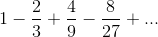None of the other answers are correct.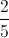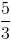Explanation:

This sum can be determined using the formula for the sum of an infinite geometric series, with initial term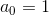and common ratio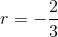: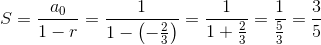### Example Question #1 : Understanding Arithmetic And Geometric Series

The fourth term in an arithmetic sequence is -20, and the eighth term is -10. What is the hundredth term in the sequence?

105

210

55

220

110

220

Explanation:

An arithmetic sequence is one in which there is a common difference between consecutive terms. For example, the sequence {2, 5, 8, 11} is an arithmetic sequence, because each term can be found by adding three to the term before it.

Let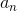denote the nth term of the sequence. Then the following formula can be used for arithmetic sequences in general: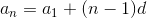, where d is the common difference between two consecutive terms.

We are given the 4th and 8th terms in the sequence, so we can write the following equations: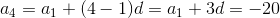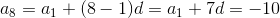.

We now have a system of two equations with two unknowns: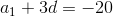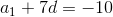Let us solve this system by subtracting the equationfrom the equation. The result of this subtraction is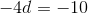.

This means that d = 2.5.

Using the equation, we can find the first term of the sequence.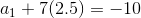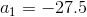Ultimately, we are asked to find the hundredth term of the sequence.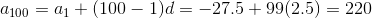### Example Question #3 : Understanding Arithmetic And Geometric Series

Find the sum, if possible: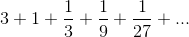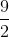Explanation:

The formula for the summation of an infinite geometric series is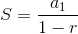,

where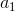is the first term in the series andis the rate of change between succesive terms.  The key here is finding the rate, or pattern, between the terms.  Because this is a geometric sequence, the rate is the constant by which each new term is multiplied.

Plugging in our values, we get: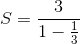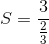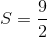### Example Question #4 : Understanding Arithmetic And Geometric Series

Find the sum, if possible: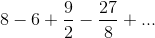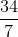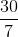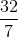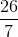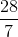Explanation:

The formula for the summation of an infinite geometric series is,

whereis the first term in the series andis the rate of change between succesive terms in a series

Because the terms switch sign, we know that the rate must be negative.

Plugging in our values, we get: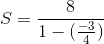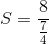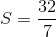### Example Question #5 : Understanding Arithmetic And Geometric Series

Find the sum, if possible: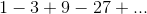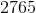No solution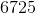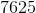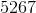No solution

Explanation:

The formula for the summation of an infinite geometric series is,

whereis the first term in the series andis the rate of change between succesive terms in a series.

In order for an infinite geometric series to have a sum,needs to be greater thanand less than, i.e.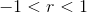.

Since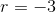, there is no solution.